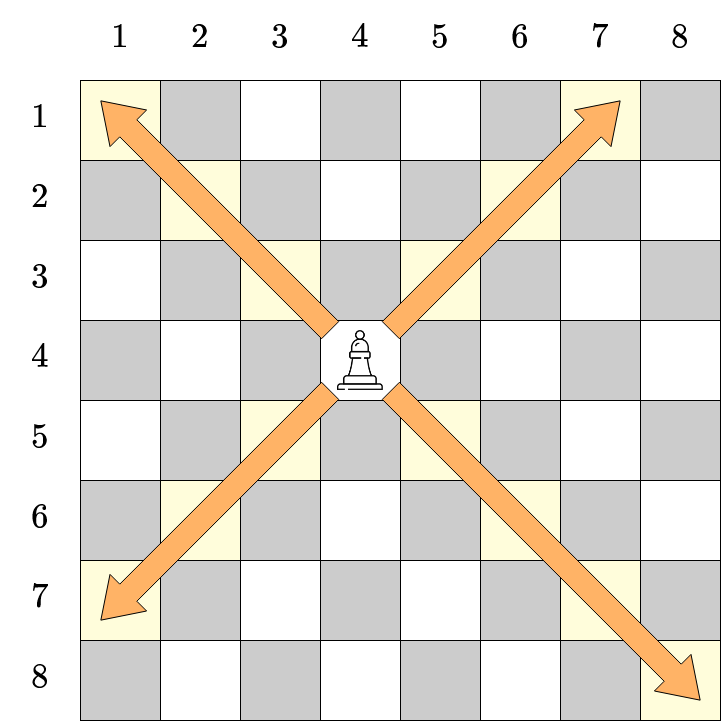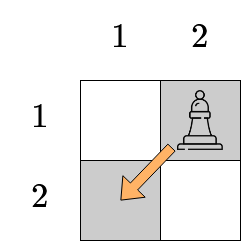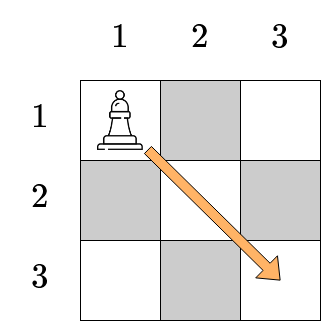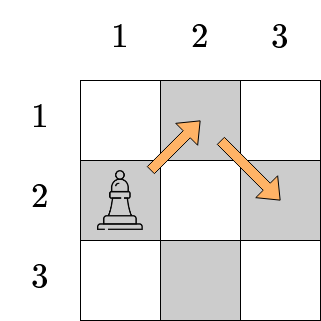## Bishop

View as PDF

Points: 0.20 (partial)
Time limit: 1.0s
Memory limit: 256M
Input: stdin
Output: stdout

Problem type
Allowed languages
C, C++, Java, Kotlin, Pascal, PyPy, Python, Scratch

Chess, sometimes called international chess, is a two-player board game, and is one of the most popular board games today. Little Neko really wants to learn Chess, however there are ~6~ different pieces, each with a movement pattern of its own! Little Neko is confused, so today let us help him learn the bishop chess piece, however on an arbitrarily sized ~n \times n~ board.

A chessboard of size ~n \times n~ is a grid board with ~n~ rows and ~n~ columns. The rows are numbered ~1~ to ~n~ from top to bottom, and the columns are numbered ~1~ to ~n~ from left to right. The square in row ~r~ and column ~c~ is denoted by the tuple ~(r, c)~.

The bishop is a chess piece that can be moved diagonally on the chessboard. Specifically, if there is a bishop in square ~(i, j)~, we can move it to square ~(i', j')~ satisfying ~|i - i'| = |j - j'|~.Illustration of bishop movement at square ~(4, 4)~. Squares in yellow are those that the piece can reach in one move.

To familiarize himself with the piece, Little Neko needs to solve the following exercise. On our chessboard, currently there is a single bishop at square ~(i, j)~. Determine if it's possible for that bishop to reach the square ~(x, y)~ using some (possibly zero) moves. If yes, find a way from square ~(i, j)~ to square ~(x, y)~ with the least number of moves. Practice makes perfect, so Little Neko needs to practice ~q~ different exercises. However Little Neko is procrastinating again, so it's up to you (again) to help him do this exercise.

#### Input

The first line contains two integers ~n~ and ~q~ (~1 \le n \le 10^9~, ~1 \le q \le 10^5~), which are the size of the chessboard, and the number of exercises, respectively.

The next ~q~ lines, each line contains four integers ~i~, ~j~, ~x~ and ~y~ (~1 \le i, j, x, y \le n~), with ~(i, j)~ denoting the square the bishop is at, and ~(x, y)~ the square it needs to move to.

#### Output

For each exercise, if there is no way to get the bishop from square ~(i, j)~ to square ~(x, y)~, output ~-1~.

Otherwise, output a single integer ~s~ on a single line, the least number of moves needed to move the bishop. After that, output ~s~ lines. On the ~t~-th line, output two integers ~r~ and ~c~, denoting the square that the bishop should move to on the ~t~-th move.

If there are multiple answers, output any.

#### Scoring

• Subtask 1 (~15~ points): ~n \le 2~

• Subtask 2 (~15~ points): ~n \le 8~

The total score for this problem is ~50~.

#### Sample Input 1

2 4
1 1 1 1
1 1 2 2
2 1 2 2
1 2 2 1


#### Sample Output 1

0
1
2 2
-1
1
2 1


#### Sample Input 2

3 5
1 1 3 3
2 1 2 3
1 2 3 2
2 2 1 1
2 2 1 2


#### Sample Output 2

1
3 3
2
1 2
2 3
2
2 1
3 2
1
1 1
-1


#### Notes

In the last exercise of the first sample, Little Neko can make the following move:In the first exercise of the second sample, Little Neko can make the following move:In the second exercise of the second sample, Little Neko can make two moves as shown below. Another valid answer is moving the bishop to ~(3, 2)~ first, then to the finishing square.